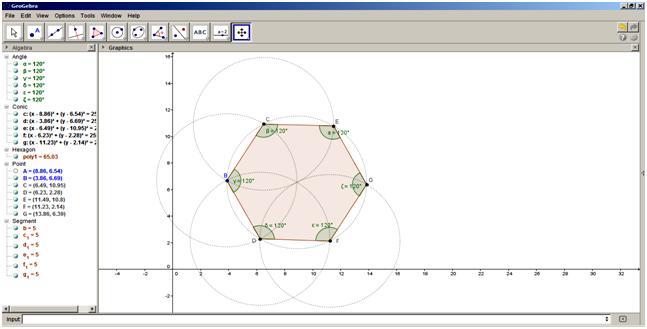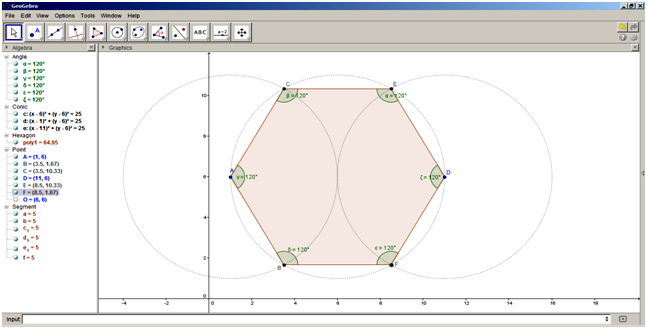## ICT PRACTICALS

### P8

SSC BOARD EXAM ICT Practical P8 - Using Geogebra draw Construct a regular polygon with six sides using the Polygon tool.

Practical Name: Using Geogebra draw
Construct a regular polygon with six sides using the Polygon tool.

Tools/Software: Computer with Geogebra Software installed.

Procedure:
Method 1
1)          Start the Geogebra Software
2)         Click down arrow on Tool No 5 and select “Circle with Centre through point.” Click in the centre of the Graphics view, and draw a circle {c} with centre at point A and passing through point B.
3)         Similarly, draw a circle {d} with centre as point B and passing through point A.
4)         Click on the Intersect two objects tool and click on circle {c} and circle {d} to intersect the two circles, you will get two vertices C and D.
5)         Then draw two circles {e} & {f} with centre as point C and centre as point D both passing through point A.
6)         Click on New point tool and click at the intersection of the circles {c} and {e} to get the vertex E and at the intersection of the circles c and f to get vertex F.
7)         Draw a circle {g} with centre as point F and passing through A.
8)         Using the New point tool, click at the intersection of the circles {c} and {g} to get the vertex G.
9)         In the Algebra area, right click the conic group and select Object properties, which will open a dialog box. Select the Style tab and change the Line Style to dotted option.
10)      Select the Polygon tool, and click on the vertices from E in anticlockwise direction to get a closed polygon.
11)       Hide the point A by right clicking it and turn off the show object option.
12)      Click on the Angle tool and click inside the hexagon. It will show the interior angles of the hexagon, all equal to 120 o
13)      Save the construction with a suitable file name.

Method 2
1)          Start the Geogebra Software
2)         Create three points in a straight line with distance difference of 5 cm. In the input bar, type A=(1,6)  then O=(6,6) and D=(11,6)
3)         Select the Circle with Centre through point tool and draw a circle with centre as O and passing through A.
4)         Similarly draw circle with centre as point A and point D passing through O.
5)         Intersect the new circles with middle circle using Intersect two objects tool to get new vertices C,B,E,F
6)         In the Algebra area, right click the conic group and select Object properties, which will open a dialog box. Select the Style tab and change the Line Style to dotted option.
7)         Select the Polygon tool, and click on the vertices from E in anticlockwise direction to get a closed polygon.
8)         Hide the point O by right clicking it and turn off the show object option.
9)         Click on the Angle tool and click inside the hexagon. It will show the interior angles of the hexagon, all equal to 120 o
10)      Save the construction with a suitable file name.

Result:  An Hexagon with side length of 5 cm. is drawn.Using Method 1Using Method 2